# How to Calculate Lot Size in Forex? – Calculation Examples, Video & Lot Size Calculator

For a foreign exchange (forex) trader, the trade size or position size decides the profit he makes more than the exit and entry points while day trading forex. Even if the trader has the best forex trading strategy, he takes too little risk or too much risk if the trade size is very small or huge. Traders should avoid taking too much risk since they will lose all their money. Some tips on how the trader should Determine Position Size are provided.

## Lot size in forex trading

A lot in forex represents the measure of position size of each trade.  A micro-lot consists of 1000 units of currency, a mini-lot of 10.000 units, and a standard lot has 100,000 units. The forex trader’s risk can be divided into account risk and trade risk. All these factors are considered to determine the correct position size, irrespective of the market conditions, trading strategy, or setup.

Now let us define a standard lot.

What is the standard lot size in forex?

The standard forex size lot is 100,000 units of currency. Usually, brokers represent forex lot size with currency units. For example, five lots are 500 000 currency units.

In this video, we will see lot size forex trading example:

## How to calculate lot size in forex?

You can calculate lot size in forex using our lot size calculator or manually using the mathematic formulas where inputs are account balance, risk percentage, and stop loss value. In the first step, the trader needs to define a risk percentage for trade and then define stop loss and a dollar per pip. In the last step, a trader must determine the currency pair’s lot size (number of units).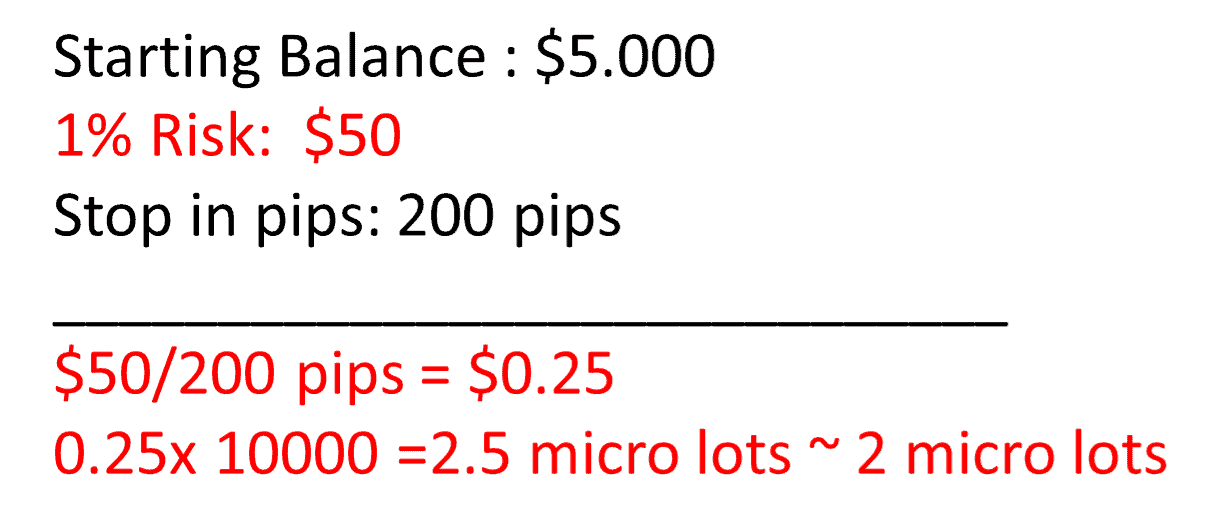## Step 1:  Calculate the risk limit for each trade

To calculate risk percentage for trade using account balance, traders can define risk in dollars per position trade.

Most traders consider specifying the dollar amount or percentage limit risked on each trade as the most crucial step in determining the forex position’s size. Lot size forex calculation is simply because professional and experienced traders will usually risk a maximum of 1% of their account in trade; usually, the amount is lower. While the other trading variables may change depending on the trade, most traders will keep the percentage they risk on the trade constantly, though the amount risked for the trade may be reduced if it exceeds the 1 percent limit.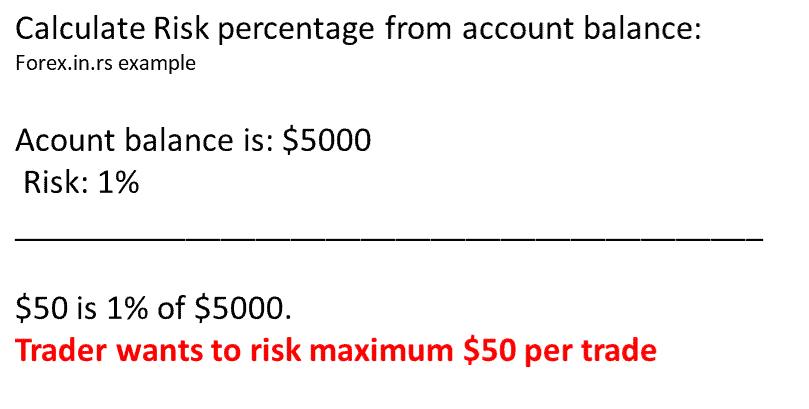(Max risk per trade position should be 1%-2%)

## Step 2:  Calculate forex size position using  dollar per pip

To calculate forex size position based on dollars per pip, traders need to divide the risk per dollar by several pips.

A pip is an abbreviation for price interest point or the percentage in point, the lowest unit for which the currency price will change. When currency pairs are considered, the pip is 0.0001 or one-hundredth of a percent. However, if the currency pair includes the Japanese yen, the pip is one percentage point or 0.01. Some brokers show prices with an additional decimal place, and this fifth decimal place is called a pipette. In the case of the Japanese yen, the third place is the pipette. m The Pip risk for each trade is calculated as the difference between the point where the stop-loss order is placed and the entry point.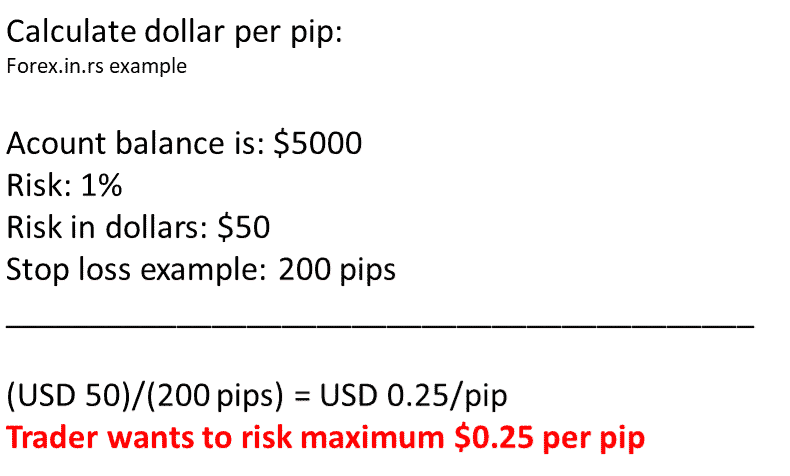A stop-loss will close a trade when it is losing a specified amount. Traders use this to ensure their loss does not exceed the account’s risk. The stop-loss level also depends on the pip risk for a specific trade. The volatility and strategy are some factors that determine pip risk. Though traders would like to ensure that their stop loss is as close to the entry point as possible, keeping it too close may end the trade before the expected forex rate movement occurs.

## How to calculate stop loss in pips?

To calculate stop loss in pips and convert it into dollars, traders need in the first step to find the difference (absolute value) between the entry price level and stop-loss price level. In the next step, traders must multiply Pips at risk, Pip value, and position size to calculate risk in dollars. For example, if a trader buys EURUSD at 1.2 and the stop-loss level is 1.195, then the stop-loss value is 50 pips (1.2-1.195=0.005=50 pips). Then, 50 Pips at risk X \$1 per Pip X 1 mini lot position size = 50 x 1 x 1= \$50.

Pips at risk= 50 pips

Pip value= for EURUSD is \$1 for 1 mini lot

Position size = 1 mini lot

Pips at risk X Pip value X position size = 50 x 1 x 1= \$50

## Step 3: Calculate forex lot size position

The second currency is called the quote currency, in a currency being traded. If the trading account is funded with the quote currency, the pip values for various lot sizes are fixed at 0.0001 of the lot size. Usually, the forex trading account is funded in US dollars. So if the quote currency is not the dollar, the pip value will be multiplied by the exchange rate for the quote currency against the US dollar.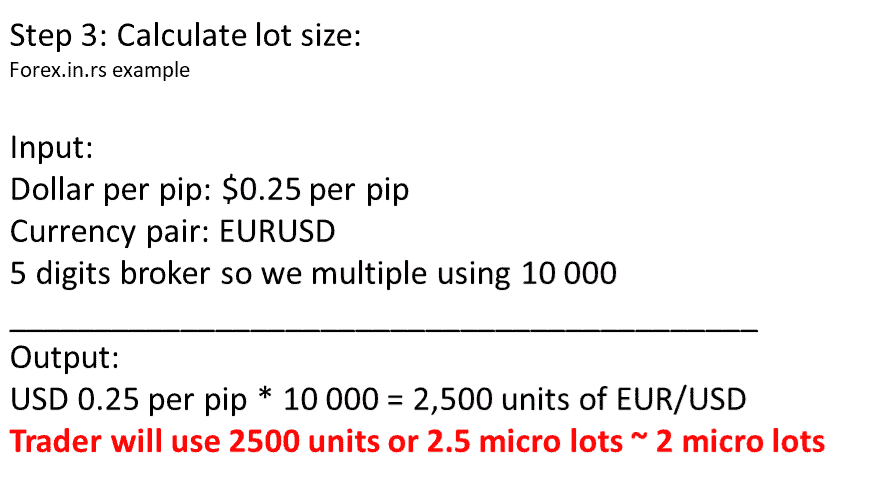What information do we need to make a forex position size calculator formula?

Let us repeat all steps once time more:

Account Currency: USD
Account Balance: \$5000, for example
Risk Percentage: 1%, for example
Stop loss: 200 pips, for example
Currency: EURUSD

How to find a lot of size in trading? In the first step, we need to calculate risk in dollars, then calculate dollars per pip, and in the last step, calculate the number of units.

Step 1: Calculate risk in dollars.
Calculate Risk percentage from account balance: 1% for \$5000 is : \$5000/100=\$50.
\$50 is 1% of \$5000.

Step 2: Calculate dollars per pip

(USD 50)/(200 pips) = USD 0.25/pip

Step 3: Calculate the number of units
USD 0.25 per pip * 10 000 = 2,500 units of EUR/USD

For five digits brokers, we use 10 000 as a multiplication. 2.5 micro lots or 0.25 mini lots is the final answer. Technically, it is two micro lots because most brokers do not allow trading less than micro-lots. In the end, here, you can use the Position Size Calculator. In MT4, calculate lot size using a lot size calculator. If you know your risk, you can calculate the lot size using the calculator below:

## Lot size calculator

The lot size forex calculator is represented below. You can use to calculate forex lot position size:

You can define the risk using either % or risk in dollars.

Latest posts by Fxigor (see all)

Fxigor

Igor has been a trader since 2007. Currently, Igor works for several prop trading companies. He is an expert in financial niche, long-term trading, and weekly technical levels. The primary field of Igor's research is the application of machine learning in algorithmic trading. Education: Computer Engineering and Ph.D. in machine learning. Igor regularly publishes trading-related videos on the Fxigor Youtube channel. To contact Igor write on: igor@forex.in.rs

Trade gold and silver. Visit the broker's page and start trading high liquidity spot metals - the most traded instruments in the world.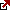Diversify your savings with a gold IRA.

Gold & silver portfolio-building assistance from product professionals.

If you want to trade stocks try: